Logarithms

 Site: TBAISD Moodle Course: Michigan Algebra II Preview 2012 Book: Logarithms Printed by: Guest user Date: Sunday, July 5, 2020, 03:03 AM

Graphing

Since logarithms and exponentials are inverse functions, the graphs will follow the inverse function rules. When graphing logarithmic functions, the values of x and y switch. Therefore, the graphs are reflections across the line y = x.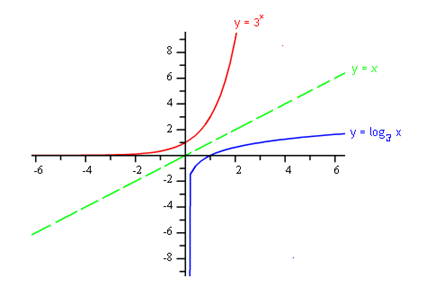Typically, when graphing a new function, it is easiest to start with a table of values. Unfortunately for logarithmic functions, it is difficult to select input values and much easier to look at the inverse function.

Example

Graph the function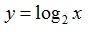Step 1. Switch the values of x and y and transform the log to exponential form.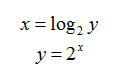Step 2. Make a table of values for the exponential function.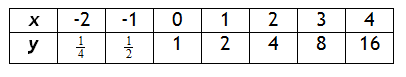Step 3. The table above is the inverse of the one needed so switch the input and output. This will create a set of ordered pairs that models the function.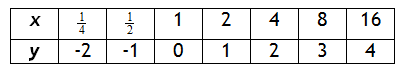Step 4. Graph the function.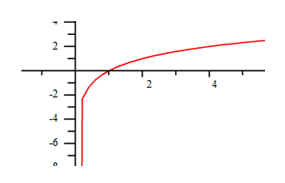Step 5. State the Domain & Range.

The x-values are approaching an asymptote at x = 0 and the y-values can be any number.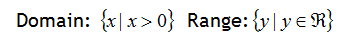Transformations

The transformations of functions that were presented in the Families of Functions unit also apply to logarithmic functions. The following list shows how the patterns apply to logarithms.

1.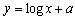translates the graph a units up; asymptote at x = 0

2.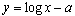translates the graph a units down; asymptote at x = 0

3.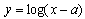translates the graph a units right; asymptote at x = a.

4.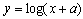translates the graph a units left; asymptote at x = -a.

5.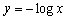reflects the graph across the x-axis; asymptote at x = 0.

6.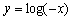reflects the graph across the y-axis; asymptote at x = 0.

Video Lesson

To learn how to graph log functions, select the following link:

Graphing Logarithmic Functions

Interactive Activity

Graphing Logarithmic and Exponential Functions

Guided Practice

To solidify your understanding of transformations of logarithmic and exponential graphs, visit the following link to Holt, Rinehart, and Winston Homework Help Online. It provides examples, video tutorials and interactive practice with answers available. The Practice and Problem Solving section has two parts. The first part offers practice with a complete video explanation for the type of problem with just a click of the video icon. The second part offers practice with the solution for each problem only a click of the light bulb away.

Practice

Graphing Log Functions Worksheet

Video Lesson:

To learn how to graph log functions, view the following video: